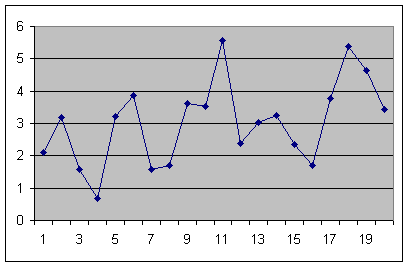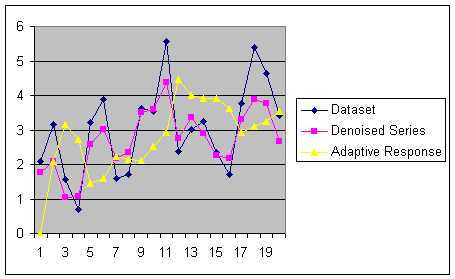# Denoising Techniques in Time-series Forecasting

Often, before being able to use a time-series, it is necessary to make it more smooth, or remove the noise that is always present in time-series. Classical approaches to this problem have always been to apply a moving average or an exponential moving average to the time-series to obtain the denoised or smoothed one. As you can read in this article on the effect of the moving average it is however very dangerous to use moving averages on time-series because they normally change the statistical properties of the time-series.

Another approach has recently emerged that is based on Wavelet Transforms. This method has a very interesting property that is, together with denoising and smoothing the time-series it maintains the time synchronization with the original time-series.

Let’s consider the following dataset:this could be representing a classical business time-series (demand for a particular good, price of a stock).

On this time-series we will apply an adaptive response rate exponential smoothing that is classically used to smooth the time-series and a Daubechies denoising algorithm. In the following picture you can see the graphical effect of both:As you can see from the picture the Adaptive response rate algorithm (as any moving average) is partially lagged and “late” with respect to the original time-series while the Daubechies denoising is perfectly on time.

Of course both algorithms are changing the statistical properties of the time-series as already described here.

 Forecasting Methods Holt Winter’s, Series Decomposition and Wavelet Benchmarks Time Series Forecasting Use of the Moving Average in Time-series Forecasting Forecasting Concepts Denoising Techniques Error Statistics Computational Performance Fast Fourier Transform Moving Averages Kernel Smoothing Active Moving Average Savitsky-Golay Smoothing Fractal Projection Downloading Financial Data from Yahoo Multiple Regression Digital Signal Processing Principal Component Analysis Curve Analysis Options Pricing with Black-Scholes Markowitz Optimal Portfolio Time-series preprocessing